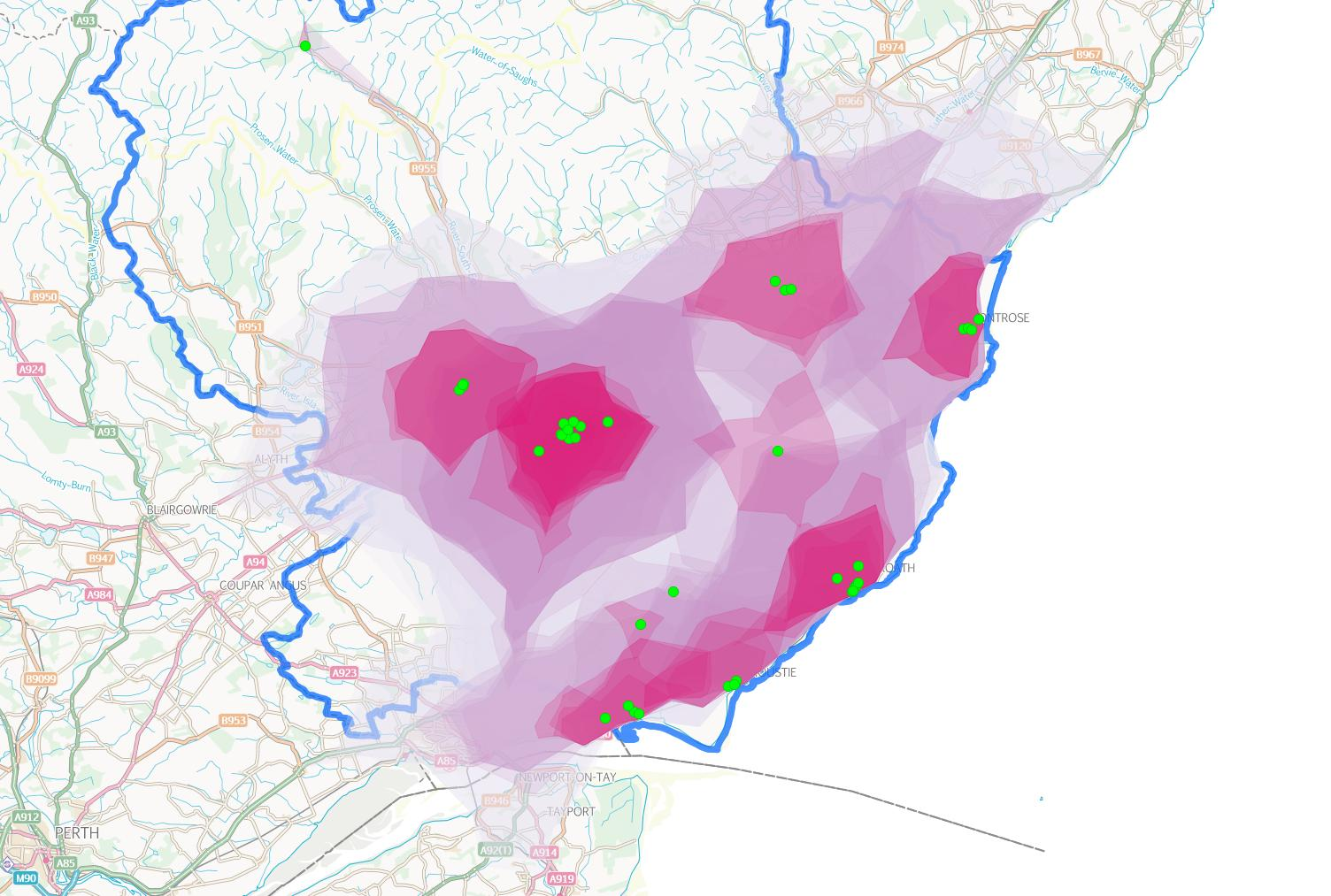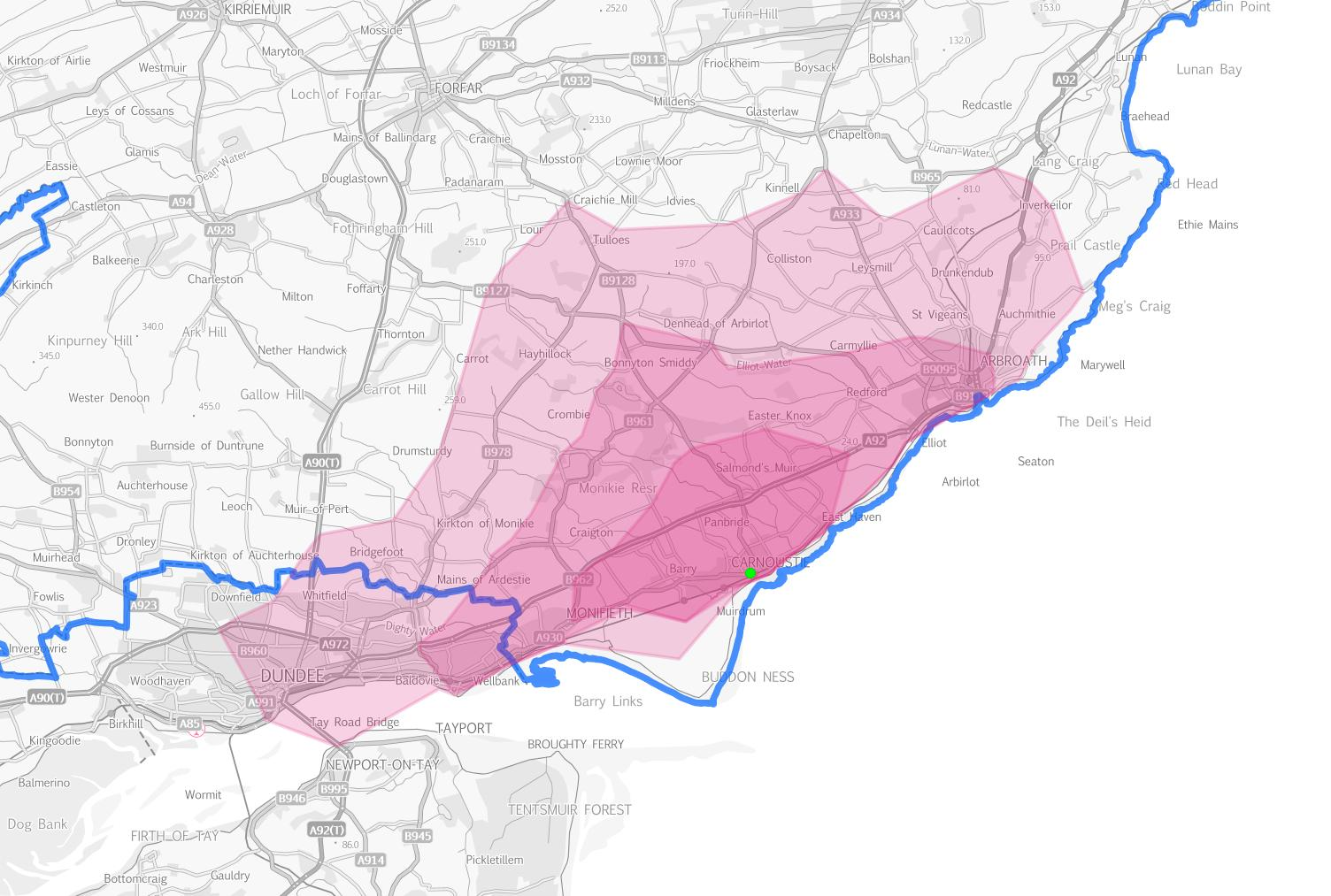# All-in-one function to create isochrones

Well, almost all-in-one. The function currently requires some prep work on the input points before being able to generate the isochrones (alphashapes). The function takes as its arguments the point input table you want the isochrones for and the time in seconds.``````CREATE OR REPLACE FUNCTION public.make_isochronesX(v_input text, v_cost integer)
RETURNS integer AS
\$BODY\$
DECLARE

cur_src refcursor;
v_nn integer;
v_geom geometry;
v_tbl varchar(200);
v_sql varchar(1000);

BEGIN
-- Drop the table being created if it exists
v_sql:='DROP TABLE IF EXISTS public.'||v_input||'_iso_'||v_cost;
EXECUTE v_sql;

-- Create the table to hold the data if it doesn't exist
v_sql:='CREATE TABLE IF NOT EXISTS public.'||v_input||'_iso_'||v_cost||'
(
id serial NOT NULL,
geometry geometry(Polygon,27700),
CONSTRAINT '||v_input||'_iso_'||v_cost||'_pkey PRIMARY KEY (id)
);
CREATE INDEX '||v_input||'_iso_'||v_cost||'_geometry
ON public.'||v_input||'_iso_'||v_cost||'
USING gist
(geometry);';
EXECUTE v_sql;

-- Drop then recreate temporary node table used in generating isochrones
DROP TABLE IF EXISTS node;
CREATE TEMPORARY TABLE node AS
SELECT id,
ST_X(geometry) AS x,
ST_Y(geometry) AS y,
geometry
FROM (
SELECT source AS id,
ST_Startpoint(geometry) AS geometry
FROM network
UNION
SELECT target AS id,
ST_Startpoint(geometry) AS geometry
FROM network
) AS node;

-- Loop through the input features, creating an isochrone for each one, and insert into the output table
OPEN cur_src FOR EXECUTE format('SELECT nearest_node FROM '||v_input);
LOOP
FETCH cur_src INTO v_nn;
FROM (
SELECT ST_Makeline(points ORDER BY id) AS openline
FROM (
SELECT row_number() over() AS id, ST_MakePoint(x, y) AS points
FROM pgr_alphashape('
SELECT *
FROM node
JOIN
(SELECT * FROM pgr_drivingDistance(''
SELECT gid AS id,
source::int4 AS source,
target::int4 AS target,
cost_time::float8 AS cost,
rcost_time::float8 AS reverse_cost
FROM network'',
'||v_nn||',
'||v_cost||',
true,
true)) AS dd ON node.id = dd.id1'::text)
) AS a
) AS foo INTO v_geom;

-- Set the table name
v_tbl:='public.'||v_input||'_iso_'||v_cost;

-- Insert the isochrone geometries into the table
EXECUTE format('
INSERT INTO %s(geometry) VALUES (\$2)', v_tbl)
USING v_tbl, v_geom;

END LOOP;
RETURN 1;
CLOSE cur_src;
END;
\$BODY\$
LANGUAGE plpgsql VOLATILE
COST 100;
``````

Note that you'll need to adjust the schema name (public) to suit your setup. You run the function with the following command:

``````SELECT public.make_isochronesX(input_table, 300);
``````

This takes your input table and generates an output table called input_table_iso_300 which will hold the 300 second isochrone polygons. If you add more points to your input table and re-run the function it overwrites the existing polygons. If you change the travel time, say to 600, then it creates a new table - input_table_iso_600.Note 1: There is a bug in the pgr_drivingdistance/pgr_alphashape code that causes the database process to crash spectacularly (don't do this in your production one) if there are less then three points in the result from which to create a polygon. The developers are working on it as I type.

Note 2: As ever this could probably be done better even though I scoured the finest code stackexchange and stackoverflow have to offer.